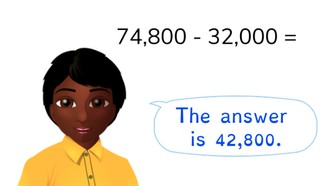Subtraction to 100,000 with simple numbers

# Subtraction to 100,000 with simple numbers

Students learn to subtract to 100,000 with simple numbers.

No account needed.8,000 schools use Gynzy92,000 teachers use Gynzy1,600,000 students use Gynzy

## General

Students learn to subtract to 100,000 with simple numbers.

## Standards

CCSS.Math.Content.4.NBT.B.4

## Learning objective

Students will be able to subtract to 100,000 with simple numbers.

## Introduction

Have the students first determine the size of the jumps by using the two numbers that have been filled in. Then fill in the other numbers.

## Instruction

Explain that to subtract to 100,000 with simple numbers, you can split the second number into ten-thousands numbers and thousands numbers. Then you subtract them from the first number in parts. In the given problem, you do this by first subtracting 20,000 and then 8,000. Show that you can also use the number line to subtract the second number in parts. The students solve the next three problems on their own. Discuss which method they have used to solve the problems. For the story problem, first have the students take the problem out of the story. Then you have them split the second number into hundreds numbers and tens numbers. Then you have them subtract the second number in the first in steps. The students will solve the next two story problems on their own. Discuss which methods the students have used to solve these problems.

Check whether the students can subtract to 100,000 with simple numbers with the following question:
- Which method do you use to subtract to 100,000 with simple numbers?

## Quiz

The students first practice subtracting to 100,000 with simple numbers with number problems. Then they are also given story problems.

## Closing

Discuss with the students the importance of subtracting to 100,000 with simple numbers. As a closing activity, have them solve three math problems.

## Teaching tips

Students that have difficulty with subtracting to 100,000 with simple numbers can first practice subtracting to 10,000 with simple numbers.

### The online teaching platform for interactive whiteboards and displays in schools

• Save time building lessons

• Manage the classroom more efficiently

• Increase student engagement